# HSC Physics Module 6 Electromagnetism Questions with Solutions

In Year 12 Physics, Module 6 Electromagnetism can be the most difficult module. Ace your Module 6 Electromagnetism exam with these 12 must know Physics questions.

HSC Physics Module 6 consists of difficult Physics concepts such as electromagnetic induction. Hence some of the most challenging questions in the HSC Physics Exams are sourced from Module 6 Electromagnetism.

Students will either love or hate Module 6 Electromagnetism because of its conceptual difficulty.

## What type of questions are commonly asked on Module 6 Electromagnetism?

The 21 question types from the Module 6 electromagnetism syllabus are listed below:

 Key Concept Question Type Electric field Motion of charges in electric fields Magnetic field Magnetic field produced by current carrying wires Magnetic force Magnetic force on a moving chargeMagnetic force on a current carrying conductorMagnetic force between parallel conductors DC electric motor Motor effect in DC motorsThe roles of the main components in a DC motorTorque produced by a DC motorImproving torque characteristics Electromagnetic induction Magnetic fluxFaraday’s Law of InductionLenz’s Law Application of Lenz’s Law Eddy currentElectromagnetic brakingAC induction motor Back EMF Back EMF in a DC motor Generators Main components in AC and DC generatorsEMF in AC and DC generatorsMaximising EMF in AC or DC generators Transmission Power loss in electrical transmissionTransformers

Test your exam readiness with the following 12 challenging exam-style questions on Module 6 Electromagnetism.

## 12 Must Know Questions on HSC Physics Module 6 Electromagnetism

### Question 1 (Motion of charged in electric fields)

Two equally charged particles travelling with the same velocity enter a region in which there is a constant magnetic field directed out of the paper as shown.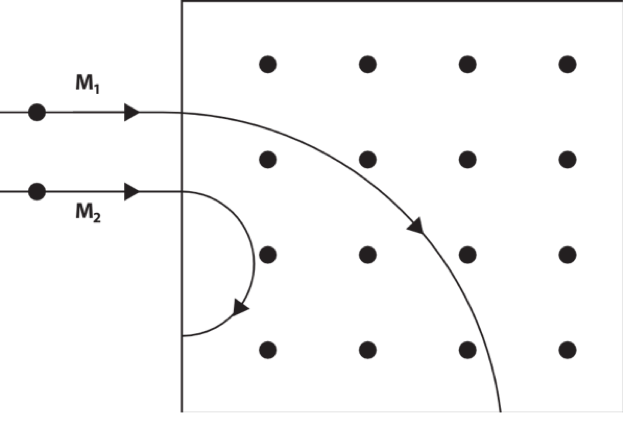The particles, ​ and ​, follow different paths in the magnetic field. Which of the following statements is correct regarding the two charged particles M1 and M2?

 (A) M1 has a smaller mass than ​M2. (B) ​M1 has a greater acceleration than ​M2 (C) M1 and M2 are both negatively charged particles (D) M1 has a greater mass to charge ratio than M2

See Question 1 solution

### Question 2 (Magnetic force between parallel conductors)

$P, Q$ and $R$ are straight, current-carrying conductors. They all carry currents of the same magnitude $I$. Conductors $P$ and $Q$ are fixed in place. The magnitude of the force between conductors $Q$ and $R$ is $F$ newtons.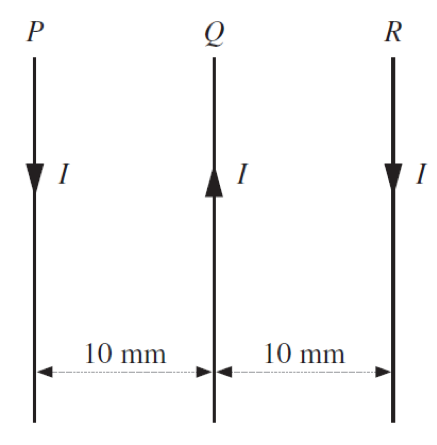What is the net force on conductor $P$ when it is in the position shown?

 (A) $\frac{F}{2}$ newtons to the left (B) $\frac{F}{2}$ newtons to the right (C) $2F$ newtons to the left (D) $2F$ newtons to the right

See Question 2 solution

### Question 3 (Motor effect in DC motors)

The diagram below shows the side view of the coil in a single coil motor.The equations $\tau=nIAB sin \theta$ and $F=ILB sin \phi$ can be used to calculate the torque in the motor and the force on the length of wire running into the page respectively.

What are the angles $\theta$ and $\phi$ in the above equations for the motor as shown in the diagram?

 (A) $\theta = 30 \degree$ $\phi = 90 \degree$ (B) $\theta = 30 \degree$ $\phi = 30 \degree$ (C) $\theta = 60 \degree$ $\phi = 90 \degree$ (D) $\theta = 60 \degree$ $\phi = 30 \degree$

See Question 3 solution

### Question 4 (Torque produced by a DC motor)

The diagram shows a DC motor with curved magnets and a constant current flowing to the rotor.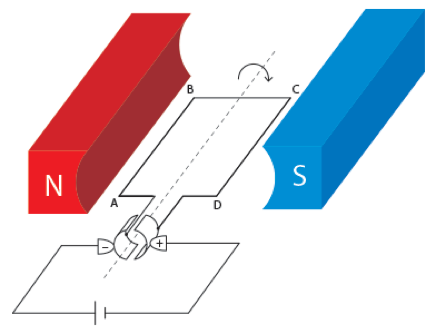Which pair of graphs best describes the behaviour of the force  on wire AB, and the torque  on the rotor as functions of time t?

 (A)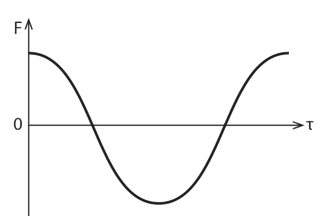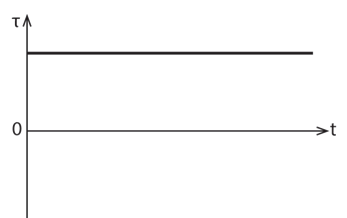(B)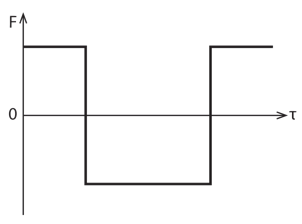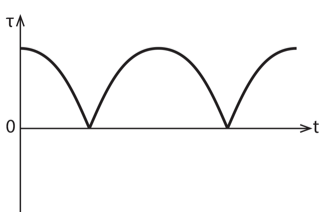(C)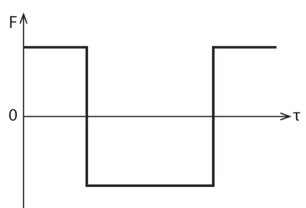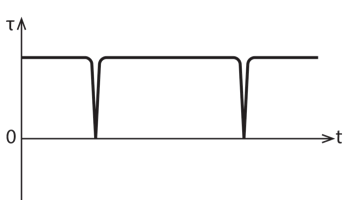(D)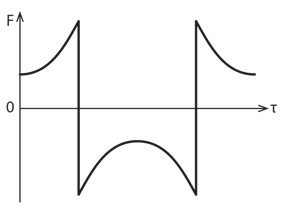See Question 4 solution

### Question 5 (Operation of DC electric motor)

The diagram shows a simple DC motor rotating clockwise in a parallel magnetic field. It consists of 100 turns of wire formed into a rectangular coil of dimensions 0.2 m x 0.1 m (AB = 0.2 m and BC= 0.1 m). The coil is in a magnetic field of 2.0 x 10-2 T. A current of 4.0 A flows through the coil.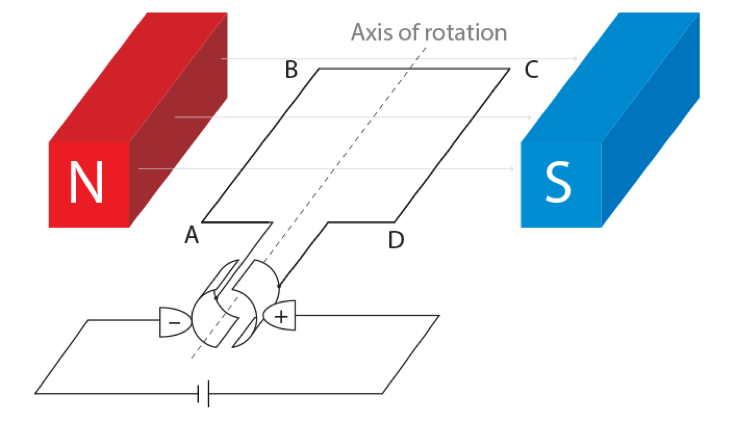(a) Describe the role of the commutator 2 (b) Calculate the magnitude and direction of the maximum torque produced by the DC motor 2 (c) A mass is attached to the coil to prevent it from rotating. Which side of the coil (AB or DC) should the mass be attached?  Hence calculate the mass. 3 (d) A student suggests the following improvements for increasing the speed of rotation of the coil. Justify the suggestions.Replace the single coil of the motor with several turnsReplace the permanent plane magnets with radial magnets 2

See Question 5 solution

### Question 6 (Faraday’s Law of Induction)

Jackie and Jim are studying electromagnetic induction. They have a small permanent magnet and a coil of wire wound around a hollowcylinder as shown in Figure 1.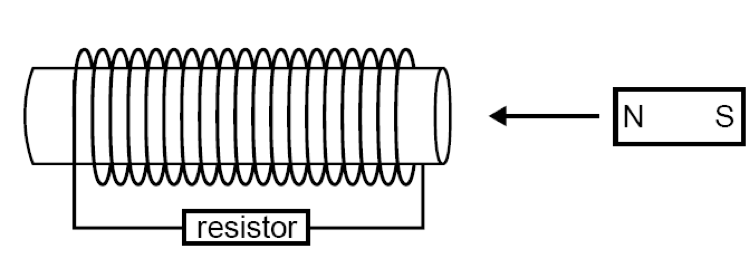Jackie moves the magnet through the coil in the direction shown at constant speed.

 (a) Indicate on the diagram the direction of the induced current that flows in the resistor. Explain the physics reason for your choice 2 (b) They next decided to move the magnet, at a constant speed, all the way through the coil and out the other side. Sketch a graph of current through the coil vs time as magnet passes through the coil.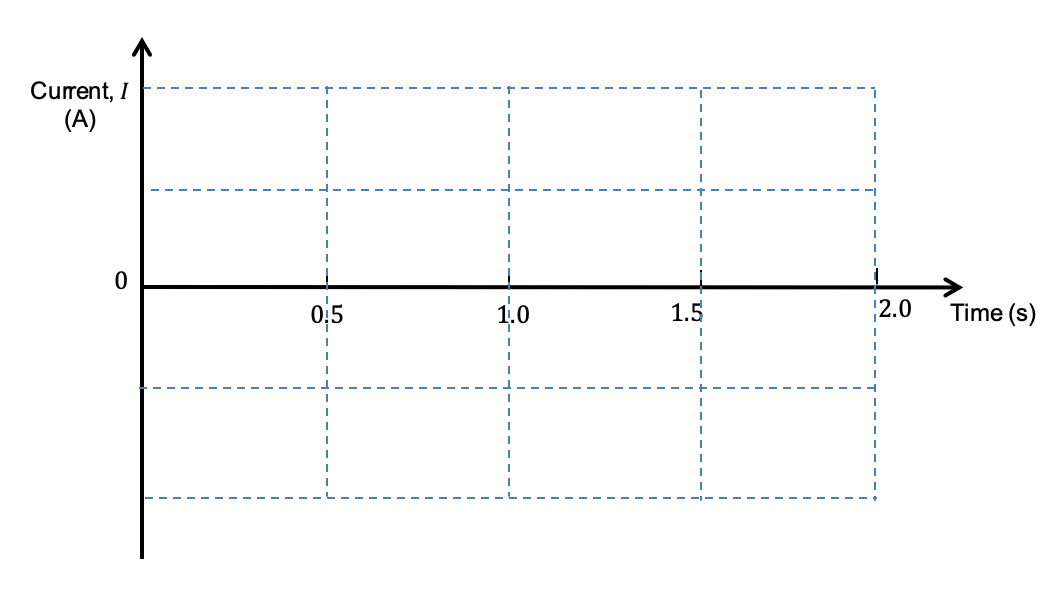2

See Question 6 solution

### Question 7 (Eddy current)

An aluminium sheet was dropped and falls through a uniform magnetic field produced a U-shaped magnet. Which diagram correctly indicates the direction of the induced current as the aluminium sheet enters the uniform magnetic field?

(A)

###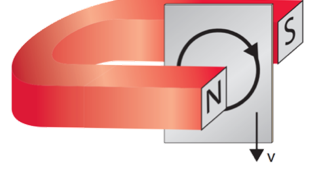(B)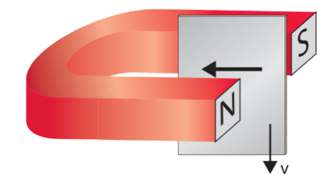(C)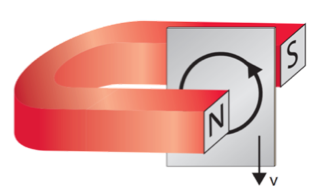(D)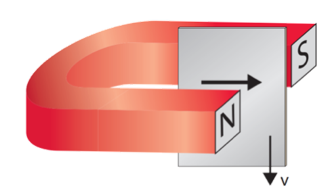See Question 7 solution

### Question 8 (Eddy current)

In a student experiment, a bar magnet is dropped through a long plastic tube of length $l$ and diameter $d$. The time taken for it to hit the floor is recorded. The experiment is repeated using a copper tube of the same length and diameter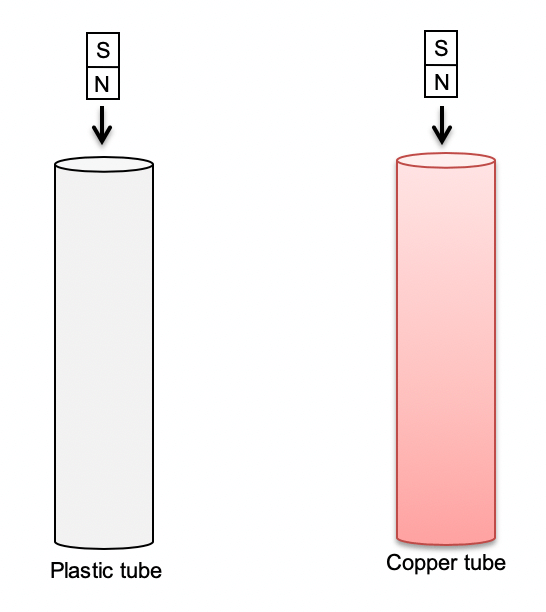Observation: The magnet takes longer to fall through the copper tube than through the plastic tube.Account for the difference in time taken for the magnet to fall. 4

See Question 8 solution

### Question 9 (Back EMF in a DC motor)

An ideal DC motor is connected to a fixed voltage DC supply. Which of the following best represents how the net EMF and the torque in the rotor coil varies with the rotational speed of the motor?

 EMF vs Speed Torque vs Speed (A)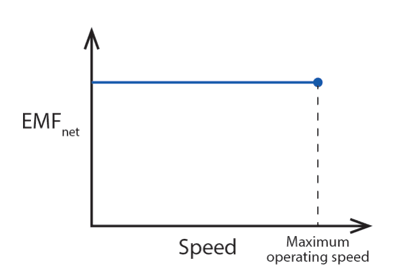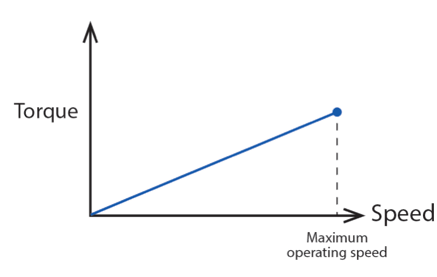(B)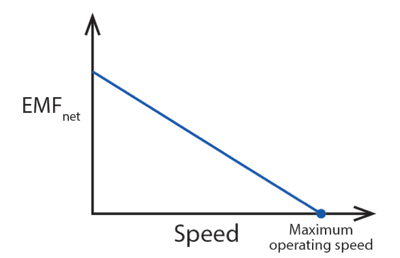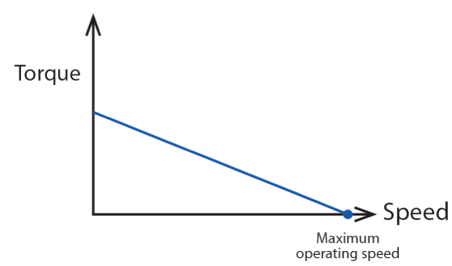(C)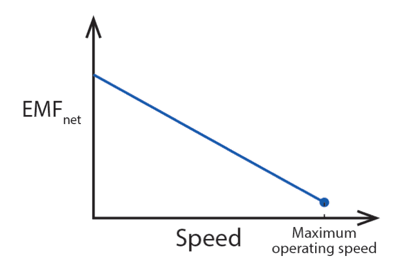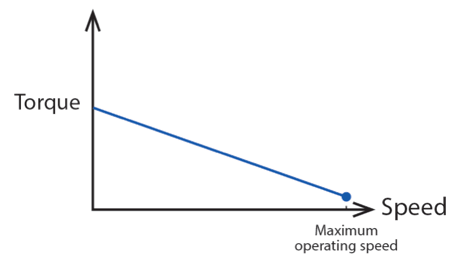(D)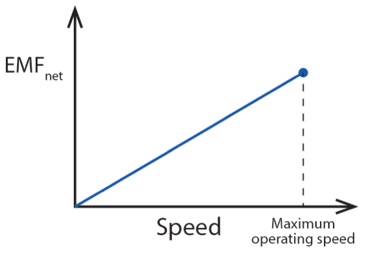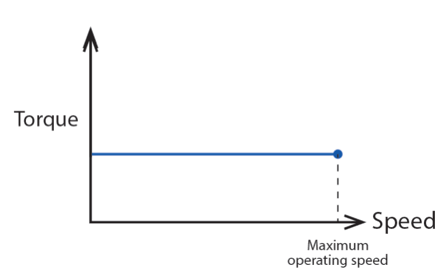See Question 9 solution

### Question 10 (Generators)

The diagram shows a generator circuit connected with a switch and light bulb.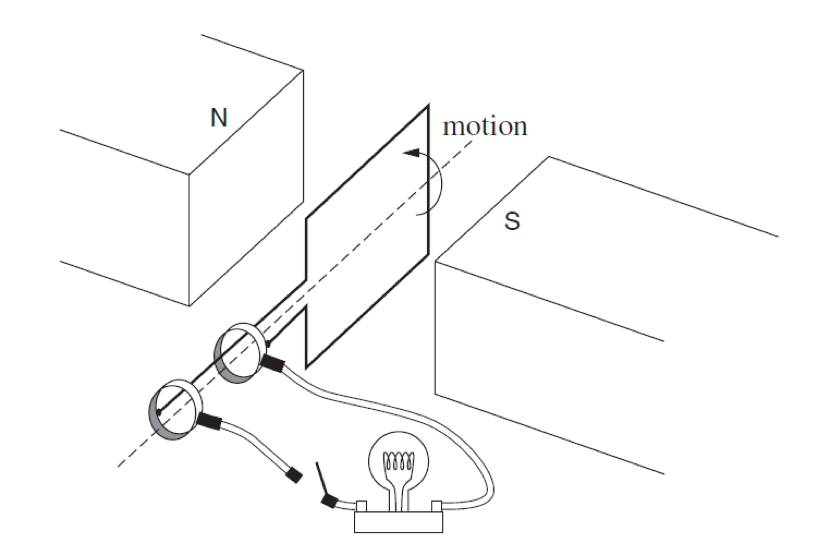Initially the coils are in the vertical positionas shown in the diagram.

The generator is rotated by one revolution in the first second with the switch closed. It is then rotated by two revolutions in the next second.

 (a) Identify the component that makes this an AC generator. Give a reason for your answer. 2 (b) Comment on the brightness of the light bulb when the switch is closed. 1 (c) Sketch a graph of current vs time for the two seconds.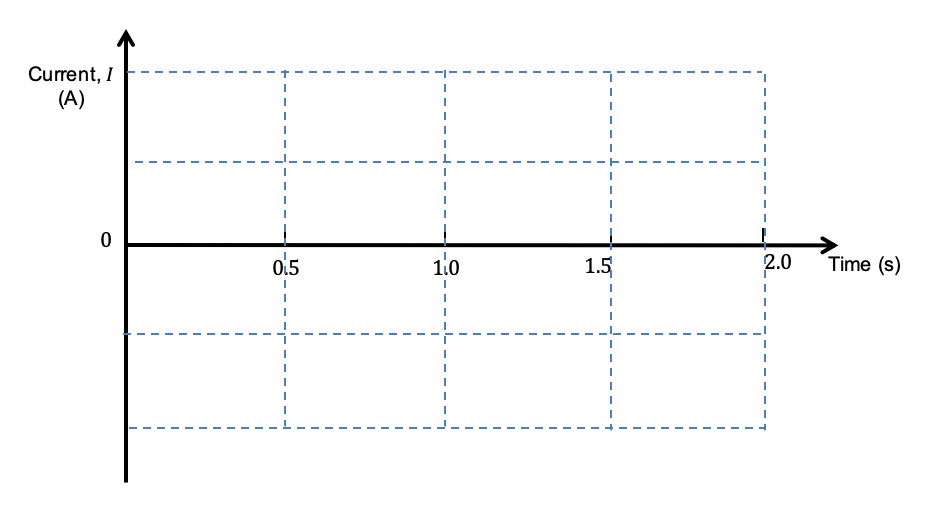2 (d) State and explain ONE modification you would make to the Generator that will result in production of a steadier EMF. 2

See Question 10 solution

### Question 11 (Generators)

In one design of a generator, a powerful magnet rotates on an axle at a frequency of 10 Hz between two fixed coils connected in series as shown below.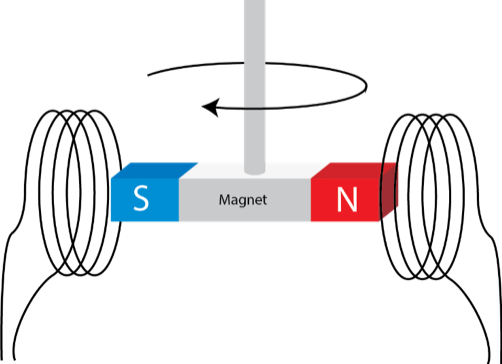(a) Is the output voltage AC or DC? Give a reason. 2 (b) Sketch the output voltage vs. time for two rotations.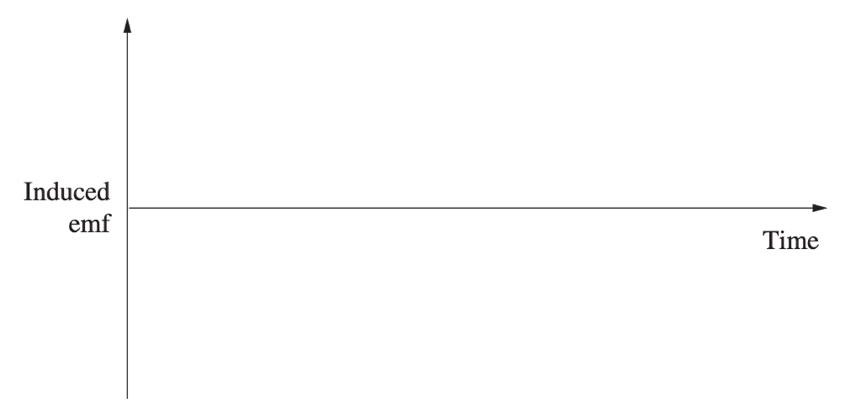2

See Question 11 solution

### Question 12 (Transformers)

A generator at a coal fired power station produces 700 MW of electrical power at 23kV. A transformer is used to increase the voltage to 330 kV.

 (a) Complete the design of a transformer by determining the following: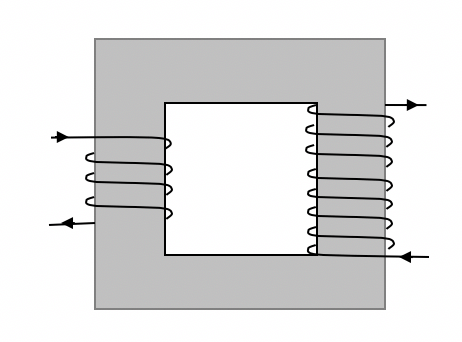The type of a transformerRatio of the number of turns in the primary and secondary coils 2 (b) Hence calculate the secondary current. 2 (c) Sketch a graph of $\frac{V_p}{V_s} = \frac{N_p}{N_s}$ for an ideal transformer. 2

See Question 12 solution

## Solutions to HSC Physics Module 6 Practice Questions

 Question Solution 1 Answer (D)Given: q1 = q2 = q and v1 = v2 = vSince $r=\frac{mv}{qB} =(\frac{v}{qB}) \times v$, r is directly proportional to m.Therefore a larger radius means the particle possesses a larger mass. 2 Answer (A)$F_{QR}=F_{PQ}=F \text{repulsive }$ since the current passing through the conductors are the same and the distance of separation between conductors Q and R is the same. $F_{PR}= \frac{F}{2} \text{ attractive }$ since the distance between conductors P and R is double the distance between conductors Q and R.Therefore, the forces acting on conductor P are:$F$ to the left$\frac{F}{2}$ to the rightHence the net force acting on conductor P is $\frac{F}{2}$ to the left 3 Answer (C).In the formula for torque $\tau=nIAB sin \theta$ represents the angle between the normal of the coil and the direction of the magnetic field. In this case it is 60̊ (or 120); it is 90 degrees away from the angle of the coil and magnetic field.On the other hand, $\phi$ in the magnetic force formula $F=ILB sin \phi$ represents the angle the wire makes with the magnetic field – it is perpendicular to the magnetic field at all times. 4 Answer (C)The force acting on the coil AB and CD are always maximum since the current flowing through the coils is at right angles to the magnetic field. The direction of the force is reversed once every half turn by the commutator.The torque produced by the coil is given by $\tau=nIAB sin \theta$. Since radial magnets are used, the angle between the magnetic field and the perpendicular area vector is at right angles in most positions. Hence the torque is at maximum $\tau=nIAB$ at almost all orientations. 5 Part (a)In a DC motor the commutator, Reverses the direction of the current into the rotor coils once every half rotation Ensures the direction of torque remains in the same direction for continuous rotationPart (b)$\tau=nIAB sin \theta = 100 \times 4 \times (0.2 \times 0.1) \times 2.0 \times 10^{-2} sin 90 = 0.16 \ Nm$The direction of the torque produced is clockwise.Part (c)The mass should be attached on the left hand side, coil AB.$\tau_coil = \tau_mass$$8 = mg \times d$$m= \frac {0.16}{gd} = \frac{0.16}{9.8 \times 0.05}= 0.327 \ kg$Part (d)The torque increases as the number of turns of the coil increases.The torque becomes steady as due to radial magnetic field produced 6 Part (a)When viewed from the right, the current flows through the solenoid in an anti-clockwise direction.This will produce an increasing magnetic field to the right to oppose the increasing magnetic field to the left by the incoming magnet.Part (b)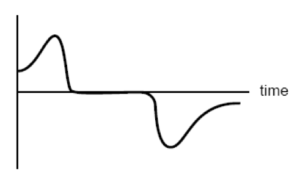7 Answer (C)When the eddy current flows in an anticlockwise direction as shown, it produces an opposing magnetic field out of the page to cancel the increasing magnetic flux the sheet experiences as it enters the magnetic field. Consequently, it will establish opposing magnetic polarities which oppose the falling motion. 8 Faraday’s Law states that an EMF is induced in a circuit when it experiences a time rate of change of magnetic flux.Furthermore, Lenz’s Law states that the direction of induced EMF is such that the current produced creates a magnetic flux that opposes the change in magnetic flux.The copper tube is conducting and will experience an EMF as the magnet falls through. Eddy currents are produced around the tube so that the magnetic flux created by them opposes the change in flux due to the falling magnet. As a result, the bottom end of the magnet is repelled upward and the top end is attracted upward, effectively slowing the magnet’s motion.The plastic tube is not conducting so it does not experience an EMF. No current is induced and thus there is no opposing magnetic flux to slow the magnet’s motion. As such, the magnet falls more quickly through the plastic tube 9 Answer (B)Refer to the flowchart for the explanation.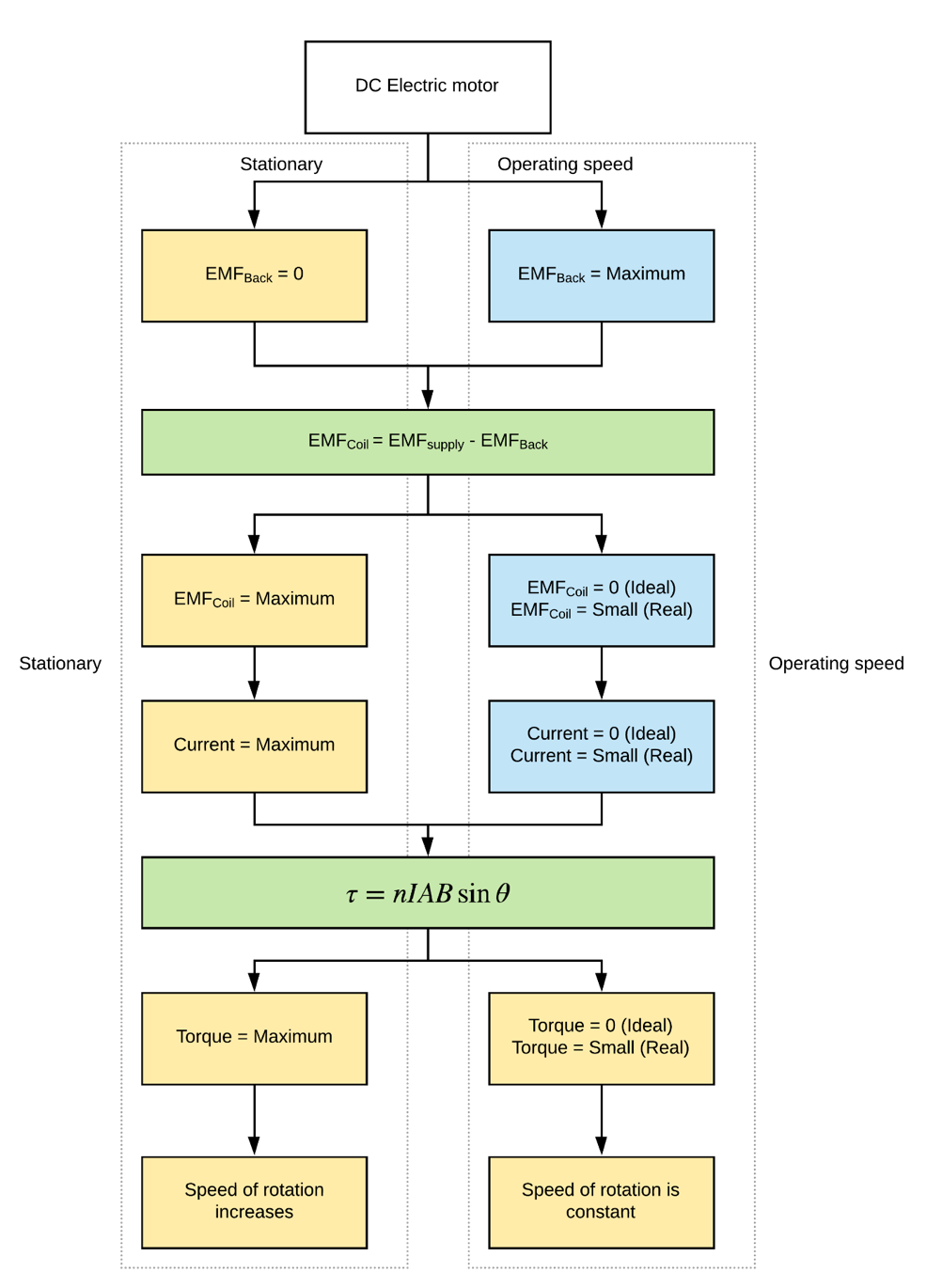10 Part (a)Slip rings.They make continuous and sliding contact with the coil without reversing the direction of the induced current in the coil.Part (b)The brightness of the light bulb flashes twice in the first revolution and four times in the second revolution with greater brightness.Part (c)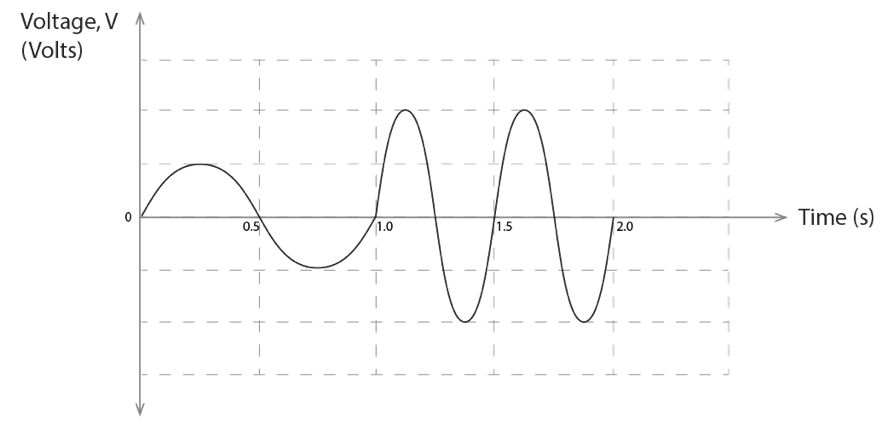Part (d)Modification: Introduce a set of perpendicular coils. OR Introduce a set of perpendicular magnets / radial magnets.Explanation:  A set of coils perpendicular to the existing coil’s orientation ensures that either coil is always experiencing a torque. Therefore, the overall torque and thus current produced by the generator will never be zero and will fluctuate less. 11 Part (a)Output voltage is AC.The direction of the induced currents switch when the polarity of the magnet reverses, therefore the output voltage is not unidirectional.Part (b)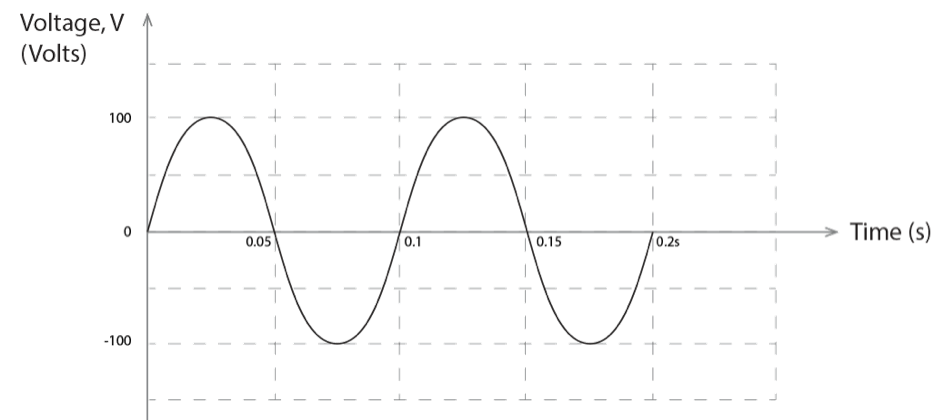12 Part (a)Step up transformer$\frac{N_p}{N_s}=\frac{V_p}{V_s} = \frac{23}{330}=0.070$ Part (b)$P=I_sV_s$$700 \times 10^6 = I_s \times 300 \times 10^3$$I_s = 2.12 \times 10^3 \ A$ Part (c)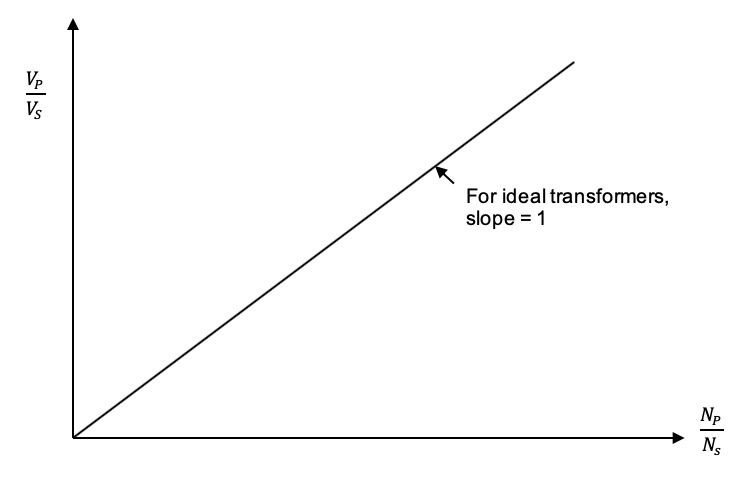## Access our library of HSC Physics Module 6 Questions

Test your understanding of any Electromagnetism concept in just 10 minutes with Learnable’s customisable quizzes with over 500+ questions for each module. Instant feedback provides immediate adjustments on your misconceptions. Try Learnable for free now.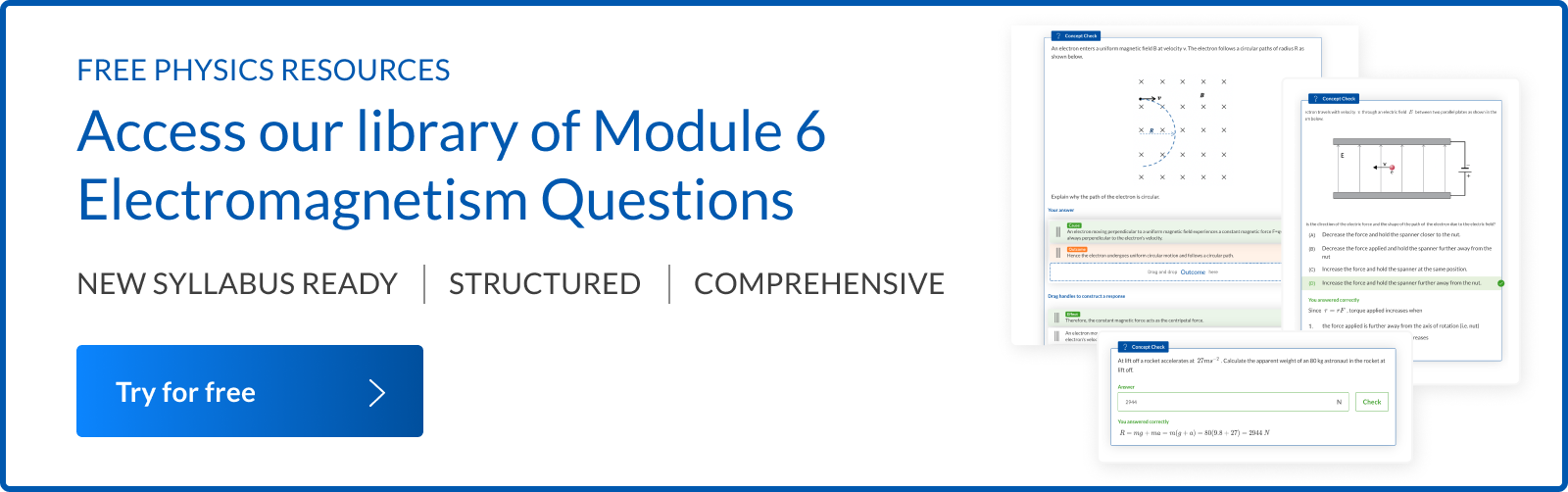### Written by DJ Kim

DJ is the founder of Learnable and has a passionate interest in education and technology. He is also the author of Physics resources on Learnable.